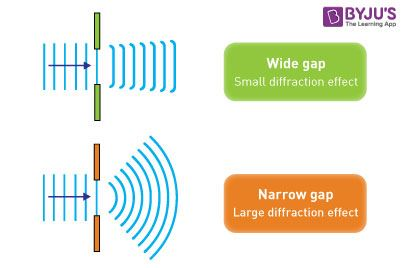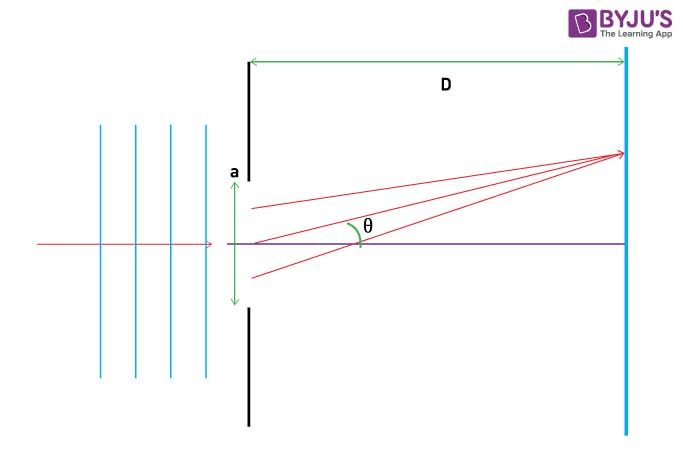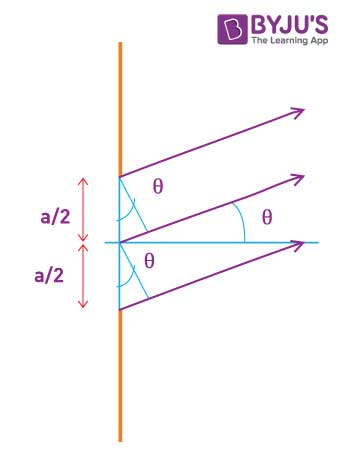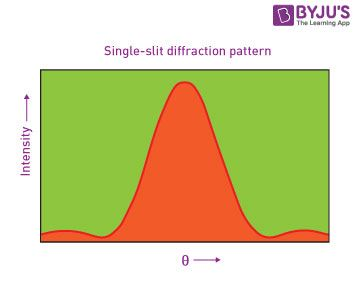# Single Slit Diffraction

When light travels in air, it encounters various phenomena like interference, refraction, reflection and diffraction. When the light comes in contact with an obstacle, diffraction of light takes place.

When light passes through a small opening, comparable in size to the wavelength λ of the light, the wavefront on the other side of the opening resembles the wave. Let us know more about the diffraction of light and single slit diffraction that occurs when light travels through a single slit. Also, let us learn what happens in a single slit diffraction experiment.

We can observe single slit diffraction when light passes through a single slit whose width (w) is on the order of the wavelength of the light. The diffraction pattern on the screen will be at a distance L >> w away from the slit. The intensity is a function of angle.

## What is Diffraction?

Diffraction of light is defined as the bending of light around corners such that it spreads out and illuminates areas where a shadow is expected. In general, it is hard to separate diffraction from interference since both occur simultaneously. The silver lining which we witness in the sky is caused due to diffraction of light. When the sunlight passes through or encounters the cloud, a silver lining is seen in the sky.
See the below video and learn about the Davisson Germer experiment and electron diffraction.When the double-slit in Young’s experiment is replaced by a single narrow slit, a broad pattern with a bright region at the centre is seen. On both sides of the centre, there are alternating dark and bright regions. The intensity becomes weaker away from the centre. In this article, we discuss the single slit diffraction of light in a detailed manner.

## What is Single Slit Diffraction?

In the single-slit diffraction experiment, we can observe the bending phenomenon of light or diffraction that causes light from a coherent source to interfere with itself and produce a distinctive pattern on the screen called the diffraction pattern. Diffraction is evident when the sources are small enough that they are relatively the size of the wavelength of light. You can see this effect in the diagram below. For large slits, the spreading out is small and generally unnoticeable.### Single Slit Diffraction Formula

We shall assume the slit width a << D. D is the separation between slit and source.We shall identify the angular position of any point on the screen by θ measured from the slit centre which divides the slit by a/2 lengths. To describe the pattern, we shall first see the condition for dark fringes. Also, let us divide the slit into zones of equal widths a/2. Let us consider a pair of rays that emanate from distances a/2 from each other as shown below.The path difference exhibited by the top two rays shown is:

 $$\begin{array}{l}\Delta L=\frac{a}{2}\sin \theta\end{array}$$

Remember that this is a calculation valid only if D is very large. For more details about the approximation check out our article on the Young’s Double Slit experiment.

We can consider any number of ray pairings that start from a distance a/2 from one another such as the bottom two rays in the diagram. Any arbitrary pair of rays at a distance of a/2 can be considered. We shall see the importance of this trick in a moment.

For a dark fringe, the path difference must cause destructive interference; the path difference must be out of phase by λ/2. (λ is the wavelength)

For the first fringe,

 $$\begin{array}{l}\Delta L = \frac{\lambda}{2} = \frac{a}{2}\sin \theta \end{array}$$ $$\begin{array}{l}\lambda = a \sin \theta\end{array}$$

For a ray emanating from any point in the slit, there exists another ray at a distance of a/2 that can cause destructive interference.

Thus, at θ = sin−1λa, there is destructive interference as any ray emanating from a point has a counterpart that causes destructive interference. Hence, a dark fringe is obtained.

For the next fringe, we can divide the slit into 4 equal parts of a/4 and apply the same logic. Thus, for the second minima:

 $$\begin{array}{l}\frac{\lambda}{2}=\frac{a}{4}\sin\theta\end{array}$$ $$\begin{array}{l}2\lambda =a\sin \theta\end{array}$$

Similarly, for the nth fringe, we can divide the slit into 2n parts and use this condition as:

 nλ = a sin θ

### The Central Maximum

The maxima lie between the minima and the width of the central maximum is simply the distance between the 1st order minima from the centre of the screen on both sides of the centre.

The position of the minima given by y (measured from the centre of the screen) is:

 tanθ≈θ≈y/D

For small θ,

sin θ≈θ

⇒ λ = a sin θ≈aθ

⇒ θ = y/D = λ/a

⇒ y = λD/a

The width of the central maximum is simply twice this value

Width of central maximum = 2λD/a

Angular width of central maximum = 2θ = 2λ/a

The diffraction pattern and intensity graph is shown below.## Frequently Asked Questions on Single Slit Diffraction

### What is phase difference?

The phase difference is defined as the difference between any two waves or the particles having the same frequency and starting from the same point. It is expressed in degrees or radians.

### What is the condition for constructive interference?

The condition for constructive interference is that the path difference should be equal to an integral multiple of the wavelength.

### What is the condition for destructive interference?

The condition for destructive interference is that the path difference should be equal to an odd integral multiple of half wavelength.

### What is temporal coherence?

Temporal coherence is known as the correlation between the field at a point and the field at the same point at a later time.

### What is the Compton effect?

Compton effect is defined as the effect that is observed when x-rays or gamma rays are scattered on a material with an increase in wavelength.

### What is total internal reflection?

Total internal reflection is defined as the complete reflection of light rays within the same medium when passing from an optically denser medium to an optically rarer medium in such a way that the angle of incidence is greater than its critical angle.

### What is fringe width?

Fringe width is defined as the distance between any two consecutive bright or dark fringes.

Stay tuned with BYJU’S to know more about diffraction of light, refraction, reflection, interference and other related concepts with the help of interactive video lessons.

Test your Knowledge on Single Slit Diffraction